Working with Cells Begin | Previous | Next Getting the Cell Value | Manipulating cells

 Getting the Cell Value To get the formatted text for a cell you can call toString method on the value returned from getValueAt. For example: `String text = grid.getValueAt( 1, 1 ).toString();` To get the unformatted value of the cell use the getCellValueAt method as in: `Object val = grid.getCellValueAt( 1, 1 );` Note that the getValueAt will return a DsCell object if there has been any cell properties set on the cell. Manipulating cells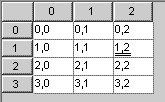There are many ways to specify cells and make changes to them. The following are similar ways to do this. The examples in the tutorial will use a variety of them. Select a cell and modify that cell. ``````grid.setRow( 1 ); grid.setCol( 2 ); grid.getCell().setFontUnderline( DsConstants.DOUBLE );``````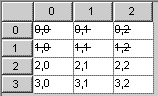Select and modify a range of cells. ``````grid.setRow( 0 ); grid.setCol( 0 ); grid.setRow2( 1 ); grid.setCol2( 2 ); grid.getCells().setFontStrikeThrough( true );``````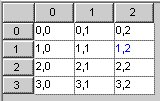Modify a cell at a given row, column without selecting it ``grid.getCellAt( 1, 2 ).setForeground( Color.blue );``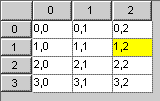Modify a range of cells without selecting them. A range of cells is specified by a bounding box by indicating the row, column of the upper left cell and the lower right cell. Those cells are also included in the range. The example below is the same ``grid.getCells( 1, 2, 2, 3 ).setBackground( Color.yellow );``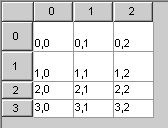Modify all cells in specified rows. The following will set the vertical alignment for all cells in rows 1 and 2. ``grid.getRows( 1, 2 ).setVerticalAlignment( DsConstants.BOTTOM );``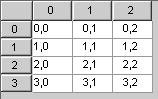Modify all cells in a specified columns. The following will set the horizontal alignment for all cells in columns 1 and 2. ``grid.getColumns( 1, 2 ).setHorizontalAlignment( DsConstants.CENTER );`` Adding formulas to cells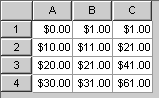Formulas (mathematical expressions) can easily be added to any cell. Over 115 functions are available to be used in the expression. The expression syntax and formulas are compatible with the leading spreadsheets on the market. ``````grid.setFormulaAt( "sum(A1:B1)", 0, 2 ); grid.setFormulaAt( "sum(A2:B2)", 1, 2 );``````